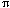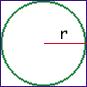Date: Mon, 24 May 1999 13:58:56 -0600 (CST)
Sender: Jose
Subject: Difficult Calculus Question

Level: Secondary

Question:
A circle is inscribed in a square. The circumference of the circle is increasing at a constant rate of 6 inches per second. As the circle expands, the square expands to maintain the condition of tangency.

find the rate at which the perimeter of the square is increasing.

find the rate of increase in the area enclosed between the circle and the square at the instant when the area of the circle is 25(pi) square inches.

 The problems of related rates usually have a quantity whose rate of change you want to find and some other quantity or quantities whose rate(s) of change you know. In this example you are asked for the rate of change of the perimetre of the square and you are told the rate of change of the circumference of the circle. Suppose that P is the perimeter of the square, C is the circumference of the circle and r is the radius of the circle.    The first step is to find a way to relate P and C. The circumference of a circle is C = 2r. From the diagram each side of the square square has length 2r and thus P = 4(2r) = 8r and hence r = P/8. Thus C = 2r = 2P/8 and thus C = (/4) P.    Both C and P change with time so differentiate both sides of the exprerssion C = (/4) P with respect to time to find a relationship between the rate of change of C and the rate of change of P. This should allow you to complete the problem.